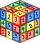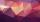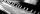# Coefficient

Determine the coefficient of this sequence: 7.2; 2.4; 0.8

Result

q =  0.333

#### Solution:Leave us a comment of example and its solution (i.e. if it is still somewhat unclear...):

Showing 0 comments:Be the first to comment!## Next similar examples:

1. QuotientDetermine the quotient and the second member of the geometric progression where a3=10, a1+a2=-1,6 a1-a2=2,4.
2. GP membersThe geometric sequence has 10 members. The last two members are 2 and -1. Which member is -1/16?
3. Six termsFind the first six terms of the sequence a1 = -3, an = 2 * an-1
4. Geometric progression 2There is geometric sequence with a1=5.7 and quotient q=-2.5. Calculate a17.
5. Geometric sequence 4It is given geometric sequence a3 = 7 and a12 = 3. Calculate s23 (= sum of the first 23 members of the sequence).
6. Q of GPCalculate quotient of geometric progression if a1=5 a a1+a2=12.
7. PianoIf Suzan practicing 10 minutes at Monday; every other day she wants to practice 2 times as much as the previous day, how many hours and minutes will have to practice on Friday?
8. Holidays - on poolChildren's tickets to the swimming pool stands x € for an adult is € 2 more expensive. There was m children in the swimming pool and adults three times less. How many euros make treasurer for pool entry?
9. AlgebraX+y=5, find xy (find the product of x and y if x+y = 5)
10. Expression with powersIf x-1/x=5, find the value of x4+1/x4
11. LegsCancer has 5 pairs of legs. The insect has 6 legs. 60 animals have a total of 500 legs. How much more are cancers than insects?
12. Theorem proveWe want to prove the sentense: If the natural number n is divisible by six, then n is divisible by three. From what assumption we started?
13. ExaminationThe class is 21 students. How many ways can choose two to examination?
14. TrigonometryIs true equality? ?
15. Reference angleFind the reference angle of each angle:
16. ConfectioneryThe village markets have 5 kinds of sweets, one weighs 31 grams. How many different ways a customer can buy 1.519 kg sweets.
17. BlocksThere are 9 interactive basic building blocks of an organization. How many two-blocks combinations are there?